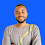2

# What's the engineering IQ picture puzzle answer?

A spanner + a  man holding helmate, wearing helmate and  2 spanners × a helmate = ?

This is one of the most tricky Math puzzle and if you don't study it carefully, you will not get the correct answer. I am going to solve step by step so that you understand better.
Read carefully to discover the correct answer to the engineering IQ puzzle bellow.

During the lockdown period, people have been exercising their skills by sharing fun and interesting games on different social media platforms. Besides spending their time doing household chores, people seek something new in their life to break the monotony of their daily routine. From dare games, push-up challenges, to fun riddles, puzzles, quizzes, they share everything to engage with their near and dear ones.

A spanner + a  man holding helmate, wearing helmate and  2 spanners × a helmate = ?

The engineering IQ puzzle is one of the puzzle going viral on the internet, many people attemting and failing especially anti matheticians.
Today I will will solve it and give the correct answer.

Here is the IQ puzzle on picture below

From the picture above, I will extract four equations from it and solve separately. In the fourth equation, I will input the values of the variables.

Let's go

Equation one:
2 spanners + 2 spanners + 2 spanners = ?
Implies 6 spanners = 30.
Spanner = 30 ÷ 6
Spanner = 5.

Equation two:

Man + Man + 10 = 20.
Implies 2men = 10,
Implies Man = 5.

Equation  three:

2 helmate + 2 helmate+ 5= 13
4 helmate  = 8
Helmate = 2.

Equation four, final equation.

A spanner + a  man holding helmate, wearing helmate and  2 spanners × a helmate =?

From the solving above,
Spanner = 5
Man = 5
Helmate = 2.

I will substitute in the final equation
5 + ( 5 + 2 +2 + 5+5)×2 =?
According to BODMAS, I will solve what is in the bracket first as thus:
5+2+2+5+5=19
So,
5+ (19) 2 =?
5+ 19×2= ?
5+ 38=?
43= ?.

Therefore, the final answer to the engineering IQ picture Puzzle is 43.

Conclusion

To get the correct answer when solving this kind of puzzle, do this First:

You have to interpret the English part of it,
Identify each equation and solve separately,
you need to apply mathematical rules carefully and solve step by step.

Also read: BODMAS and PEMDAS EXPLAINED WITH EXAMPLES

1.1.